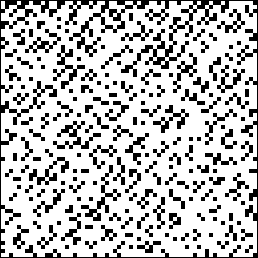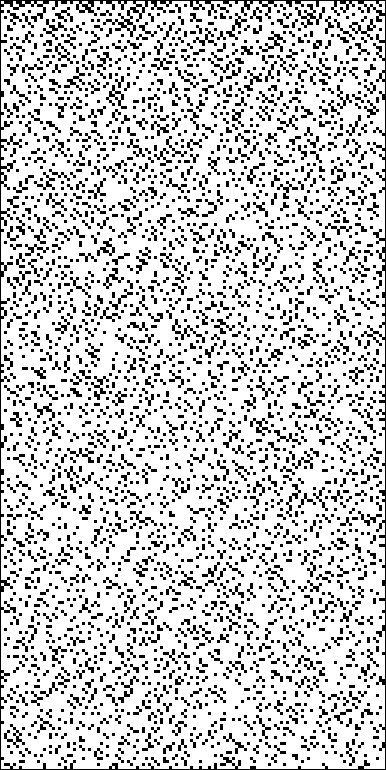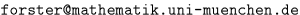## Pictures of Primes

• Primes < 8192
• Primes < 2^16
• Primes 10^50 < p < 10^50 + 2^15
• Primes 10^100 < p < 10^100 + 2^15
• Primes 10^200 < p < 10^200 + 2^15
• Primes 10^400 < p < 10^400 + 2^15
• data files

•picture of odd primes < 8192

The above picture is a matrix of 64 rows and 64 columns of altogether 4096 black or white squares. The squares are numbered (in row major order) from 0 to 4095. The n-th square is black iff the number 2n + 1 is prime. For example, the black square in the right upper corner represents the prime 127, and the black square in the right lower corner represents the prime 8191. You can observe, besides many twin primes, the two kinds of triple primes (patterns `xx_x` resp. `x_xx`) and some quadruple primes (pattern `xx_xx`).

The picture below is a 256 by 128 matrix which shows all odd primes < 2^16 = 65536.`data file`

` bigger primes `
Otto Forster (), 95-05-16/97-04-30